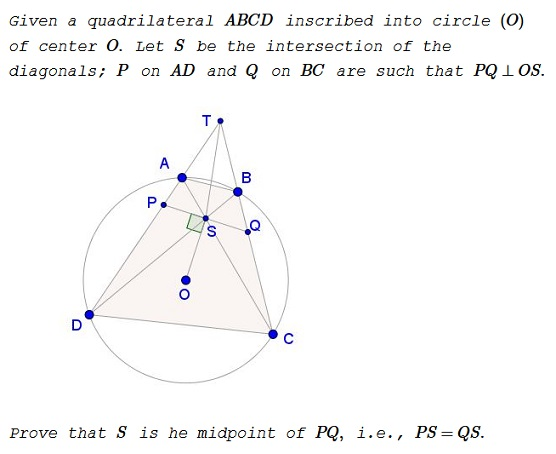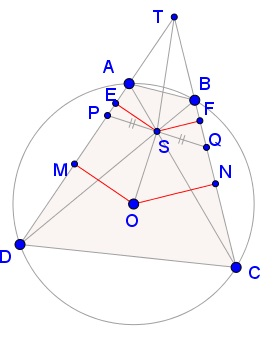# Stathis Koutras' Butterfly

### Problem### Solution

Let $EM,FN\,$ be the orthogonal projections of $SO\,$ on $AD,BC,\,$ respectively. And let $T\,$ be the intersection of $AD\,$ and $BC.\,$Since $OS\perp PQ,\,$ we may apply Stathis Koutras' theorem to obtain

(1)

$\displaystyle\frac{EM}{FN}=\frac{TQ}{TP}.$

But $EM,FN\,$ are the corresponding segments of obviously similar triangles $SAD\,$ and $SBC\,$ ($E,F\,$ are the feet of the altitudes whereas $M,N\,$ the midpoints of the corresponding sides) and, therefore, their ratio shall be equal to the ratio of the corresponding altitudes, i.e., $\displaystyle\frac{EM}{FN}=\frac{SE}{SF}.\,$ Combining this with (1),

$\displaystyle\frac{TQ}{TP}=\frac{SE}{SF}\,\Rightarrow\,TQ\cdot SF=TP\cdot SE\,\Rightarrow\,[\Delta TSQ]=[\Delta TSP],$

where $[X]\,$ denotes the area of shape $X.\,$ The two triangles share the altitude from $T,\,$ implying that $S\,$ is indeed the midpoint of $PQ,\,$ thus proving the Butterfly Theorem.

### Acknowledgment

Stathis Koutras has kindly posted at the CutTheKnotMath facebook page his novel proof of the Butterfly Theorem.

### Butterfly Theorem and Variants